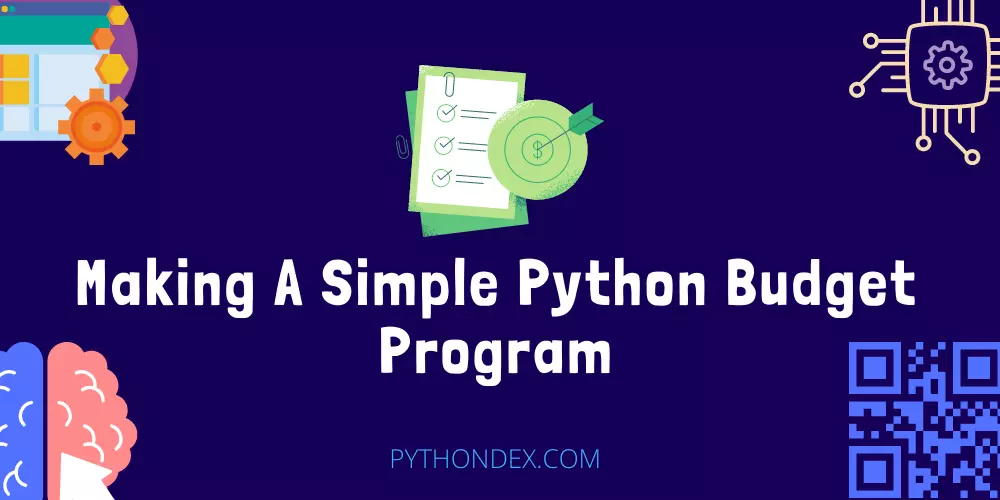ExplorePython Programs# Making A Simple Python Budget programWant to make a budget program in python, then today in this guide I will show you how to make a simple python budget program which will allow you to manage your budget with python programming.

Doing your budget is very important. If you are a programmer, then you can do your budget with python programming easily. It is very easy to do. I will show you step by step, so read this guide till the end.

What and how will this python budget program do and work

• It will tell you your total expenses
• It will calculate and tell your budget

Enough of talking now let’s see how to make this budget program in python programming with code.

## Python Budget Program Source Code

``````
import os
import sys

class Application():
def __init__(self):
self.income = 0
self.expenses = 0
self.expense_list = []
self.expense_name = []
self.income_name = []
self.income_list = []
self.prompt_income()

def income_sum(self):
self.income = sum(self.income_list)

def expense_sum(self):
self.expenses = sum(self.expense_list)

def income_check(self):
if not self.income_list:
print('Please enter atleast one source of income. ')
self.prompt_income()
else:
return

def expense_check(self):
if not self.expense_list:
print('Please enter atleast one expense. ')
self.prompt_expense()
else:
return

def prompt_income(self):
x = False
while not x:
if result == 'y':
income_input = int(input('Enter source of income. [Numbers Only]: '))
self.income_list.append(income_input)
income_name = input('Enter income name. [Name Only]: ')
self.income_name.append(income_name)
else:
self.income_check()
x = True
self.income_sum()
name = [name for name in self.income_name]
income = [income for income in self.income_list]
incomedict = dict(zip(name, income))
for k in incomedict:
print(k + ': ', '\$' + str(incomedict[k]))
print('Total user income: ', '\$' + str(self.income))
self.prompt_expense()

def prompt_expense(self):
x = False
while not x:
if result == 'y':
expense_input = int(input('Enter expense amount. [Numbers Only]: '))
self.expense_list.append(expense_input)
expense_name = input('Enter expense name. [Name Only]: ')
self.expense_name.append(expense_name)
else:
self.expense_check()
x = True
self.expense_sum()
name = [name for name in self.expense_name]
expense = [income for income in self.expense_list]
expensedict = dict(zip(name, expense))
for k in expensedict:
print(k + ': ', '\$' + str(expensedict[k]))
print('Total user expenses: ', '\$' + str(self.expenses))
self.uservalue()

def uservalue(self):
valoutput = self.income - self.expenses
if valoutput < 0:
print('You are in the negative, you have a deficit of ' + '\$' + str(valoutput))
if valoutput == 0:
print('You have broken even, you are spending exactly as much as you make.')
if valoutput > 0:
print('You are in the positive, you have a surplus of ' + '\$' + str(valoutput))
another = input('Would you like to run another analysis? [y/n]: ')
if another == 'y':
self.reset_program()
else:
self.close_program()

def reset_program(self):
self.income = 0
self.expenses = 0
del self.expense_list[0:]
del self.expense_name[0:]
del self.income_name[0:]
del self.income_list[0:]
self.prompt_income()

def close_program(self):
print('Exiting Program.')
sys.exit(0)

if __name__ == '__main__':
Application()
```
```

Above is the python code for a budget program. Copy the code and paste it into your python file and run the file in your compiler or use an online compiler.

This is a command line program below is the code output of the python budget program

Output

``````
Enter source of income. [Numbers Only]: 1000
Enter income name. [Name Only]: Job
Enter source of income. [Numbers Only]: 200
Enter income name. [Name Only]: Real estate
Job:  \$1000
Real estate:  \$200
Total user income:  \$1200
Enter expense amount. [Numbers Only]: 400
Enter expense name. [Name Only]: Groceries
Enter expense amount. [Numbers Only]: 200
Enter expense name. [Name Only]: Recharge
Groceries:  \$400
Recharge:  \$200
Total user expenses:  \$600
You are in the positive, you have a surplus of \$600
Would you like to run another analysis? [y/n]: n
Exiting Program.
```
```

First it will ask you to add your income source and income you need to type y or n you need to enter y to enter your income after that it will ask you how much is your income and what is the name of the income.

You can add as many income sources after you need to at least add one to continue after that it will ask you to enter your expenses.

Finally, it will calculate your expenses and income and tell you your budget and if you are overspending. You can create another budget report if not, it will end the program.

## Summary

So this was the python budget program. You can now track your income and expenses using python programming. I hope you liked this program.

Here are some more python programs guides you may find helpful:

I hope you found this tutorial helpful and you found what you were looking for. If you want more python tutorials like this, then do join our Telegram channel for future updates.

Thanks for reading, have a nice day 🙂